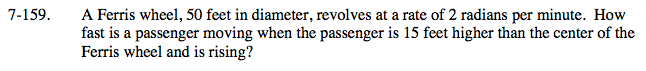### Home > CALC > Chapter Ch7 > Lesson 7.3.5 > Problem7-159

7-159.

A Ferris wheel, 50 feet in diameter, revolves at a rate of 2 radians per minute. How fast is a passenger moving vertically when the passenger is 15 feet higher than the center of the Ferris wheel and is rising? Homework Help ✎Sketch a diagram. Assume the wheel is rotating counterclockwise and let the passenger be at acute angle θ and height H above a horizontal radius. Do you see the right triangle? Write a geometric equation relating H and θ? Then implicitly differentiate the equation with respect to time.

$\frac{d\theta}{dt} =2. \ \ \ \text{You are trying to solve for }\frac{dH}{dt}.$

$h=25\sin(\theta)$

$\frac{dh}{dt}=25\cos(\theta)\frac{d\theta}{dt}$

Determine the value of cos(θ) when h = 15 ft.
Once you compute this value, use the differential equation to solve the problem.